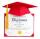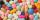Masons

1 mason casts 30.8 meters square in 8 hours. How long casts 4 masons 178 meters square?

Result

x =  11.6 h

Solution:Leave us a comment of this math problem and its solution (i.e. if it is still somewhat unclear...):Be the first to comment!Next similar math problems:

1. DiplomasSuzanne helped write out diplomas. Issuance its first took 3 minutes, each next 2.3 minutes. How much time it will take to write out n=16 diplomas (including the first), write out this pace?
2. Greg and BillGreg is 18 years old. He is 6 less than 4 times Bill's age. How old is Bill?
3. Grandfather and grandmotherThe old mother is 5 years younger than the old father. Together they are 153 years old. How many years has each of them?
4. PillsIf it takes 20 minutes to run a batch of 100 pills how many minutes would it take to run a batch of 50 pills
5. Scale of the mapDetermine the scale of the map if the actual distance between A and B is 720 km and distance on the map is 20 cm.
6. DonutsFind how many donuts each student will receive if you share 126 donuts in a ratio of 1:5:8
7. New refrigeratorNew refrigerator sells for 1024 USD, Monday will be 25% discount. How much USD will save, and what will be the price?
8. Sales offGoods is worth € 70 and the price of goods fell two weeks in a row by 10%. How many % decreased overall?
9. Percents - easyHow many percent is 432 out of 434?
10. The percentages in practiceIf every tenth apple on the tree is rotten it can be expressed by percentages: 10% of the apples on the tree is rotten. Tell percent using the following information: a. in June rained 6 days b, increase worker pay 500 euros to 50 euros c, grabbed 21 fro
11. MO 2016 Numerical axisCat's school use a special numerical axis. The distance between the numbers 1 and 2 is 1 cm, the distance between the numbers 2 and 3 is 3 cm, between the numbers 3 and 4 is 5 cm and so on, the distance between the next pair of natural numbers is always in
12. InequationSolve the inequation: 5k - (7k - 1)≤ 2/5 . (5-k)-2
13. Apples 2James has 13 apples. He has 30 percent more apples than Sam. How many apples has Sam?
14. Unknown numberIdentify unknown number which 1/5 is 40 greater than one tenth of that number.
15. Base, percents, valueBase is 344084 which is 100 %. How many percent is 384177?
16. Down syndromeDown syndrome is one of the serious diseases caused by a gene mutation. Down syndrome occurs in approximately every 550-born child. Express the incidence of Down's syndrome in newborns at per mille.
17. EquationSolve the equation: 1/2-2/8 = 1/10; Write the result as a decimal number.